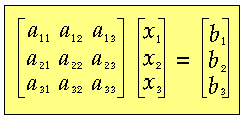# Solving Ax=b; when to use LU decomposition?

I want to solve the linear equation below:

$$Ax = b$$

For this purpose, I'm writing a C++ code. I have written both routines for decomposing A matrix to L and U matrices, and for calculating inverse of A matrix.

I may multiply both sides with A-1:

$$Ax = b$$
$$A^{-1}Ax = A^{-1}b$$
$$x = A^{-1}b$$

Or, I can use LU decomposition:

$$Ax = b$$
$$A = LU$$
$$Ax = LUx = Ly = b$$
$$Solve\,\,\,\,\, Ly = b\,\,\,\,\, for\,\,\,\,\, y$$
$$Solve\,\,\,\,\, Ux = y\,\,\,\,\, for\,\,\,\,\, x$$

LU decomposition method is said to be faster. But, I'm not sure if these rumors are true for all cases. I have a feeling that the first method (matrix inversion method) would be faster for smaller A matrices.

My question is, how do I prefer which method to use?

AlephZero
Homework Helper
LU decomposition method is said to be faster. But, I'm not sure if these rumors are true for all cases.

It is faster for any matrix of order greater than 1x1. Well, to be fair, you can write special code for a 2x2 matrix, but the amount of computation is so tiny that it's hardly worth the bother, unless you need to solve 100 million sets of 2x2 equations (and there are numerical applications that DO need to do that sort of thing.)

For at least 999 algorithms out of 1000, finding the explicit inverse of a matrix is not the fastest way, though "beginners" are often tempted to just "translate" math equations containing an inverse matrices into a programming language.

Actually, a fast and reliable way to calculate the inverse of an NxN matrix is to first find the LU decomposition, and then solve N sets of equations where the "b" vectors have one 1 and the other terms all zero, to find the columns of the inverse matrix one at a time. That is already more work than solving one set of equations using LU decomposition.

Thank you for your guidance. I will stick to LU decomposition.

Actually, a fast and reliable way to calculate the inverse of an NxN matrix is to first find the LU decomposition, and then solve N sets of equations where the "b" vectors have one 1 and the other terms all zero, to find the columns of the inverse matrix one at a time. That is already more work than solving one set of equations using LU decomposition.

This is an interesting information.
I didn't understand what b vector to choose for calculating the inverse of A. Can you please a b vector and explain the method to find the inverse of A matrix?

I like Serena
Homework Helper
Did you consider how robust the algorithms are?
An important concern is usually to minimize the result of rounding errors.
This is especially important when the matrix is close to singular.

SteamKing
Staff Emeritus
Homework Helper
To calculate the inverse of matrix A (n x n), the b matrix is equal to the n x n Identity Matrix (only 1s on the main diagonal, all zeros elsewhere).

To calculate the inverse of matrix A (n x n), the b matrix is equal to the n x n Identity Matrix (only 1s on the main diagonal, all zeros elsewhere).

In the Ax=b equation I'm working with, A is a square matrix, x and b are column vectors.

Like this:So, how do I make the b vector an nxn identity matrix?

HallsofIvy
Homework Helper
solve N sets of equations where the "b" vectors have one 1 and the other terms all zero, to find the columns of the inverse matrix one at a time.

I didn't understand what b vector to choose for calculating the inverse of A. Can you please a b vector and explain the method to find the inverse of A matrix?
AlephZero told you that. For, say, a 4 by 4 matrix, use
$$\begin{bmatrix}1 \\ 0 \\ 0 \\ 0\end{bmatrix}$$, $$\begin{bmatrix}0 \\ 1 \\ 0 \\ 0\end{bmatrix}$$, $$\begin{bmatrix}0 \\ 0 \\ 1 \\ 0\end{bmatrix}$$, and $$\begin{bmatrix}0 \\ 0 \\ 0 \\ 1\end{bmatrix}$$

Hmm. I will substitute columns of the identity matrix for b vector for n times to find n different x vectors. Next, I will merge these x vectors to form the inverse of the A matrix. Did I understand it right?

lurflurf
Homework Helper
^Yes that is right.
The LU decomposition is basically free, so even when it does not help it cannot hurt. Computing the inverse is best avoided if possible, both for speed and stability.